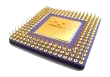CDSP-k2 Performance Benchmark suite for common DSP algorithmsThe following table presents a performance estimation for some common DSP algorithms.
The estimation is based on an architecture that features one single-cycle MAC unit, a Butterfly unit, and supports the post-increment and bit-reverse indexing facilities.

 Operation Description Conditions Parameters Cycle-Count FIR Finite Impulse Response Filter with Constant or Variable Coefficients Arbitrary number of Coefficients and Samples N=number of coefficients M=number of samples M*(N+p)+q IIR Direct Form II IIR Filter, Constant or Variable Coefficients Cascaded biquad sections, Samples multiple of 8 N=number of samples M=number of cells M*(10*N+p)+q LMS FIR Coefficients Update Update the FIR coefficients using the Least Mean Square Algorithm Even number of coefficients N=Number of coefficients 2*N+p Autocorrelation Autocorrelation Arbitrary input and output vector size N=number of input samples M=number of output samples M*(N+p)+q Energy Square each element in a vector and sum all the squared values Arbitrary vector size N=vectors size N+p FFT Radix 2 Fast Fourier Transform Number of samples is a power of 2 N=number of samples Log2(N)*(4*N/2+p)+q Dot Product Dot product of two equal-size vectors Arbitrary vector size N=vector size N+p Weighted Vector Sum Add two vectors elements having one of them multiplied by a constant Even number of elements in the vectors N=vectors size 2*N+p Max/Min Find the minimum/maximum value in a vector and retain its position Arbitrary vector size N=vectors size N+p Search/Skip Search until/while a value is found in a vector and retain the (last) position where it was found Arbitrary vector size N=vectors size N+p Move Move a vector from one position to another in memory Arbitrary vector size N=vectors size N+p

Note 1: "p" and "q" count for the overhead loop-initialization instructions. Performance significantly degrades when "N" is relatively small (<32) as compared with "p".